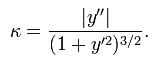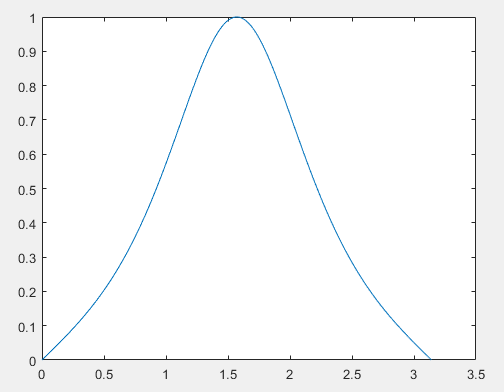• matlab曲率拟合代码LM包装 八度埃塔德（） Matlab例程，用于拟合线性正向/反向模型/ CCA。 需要Matlab R2019b或更高版本（已在R2019b和R2020a / b上测试）。 介绍 该软件包包含用于拟合线性正向/反向模型（反卷积）...
• Matlab平均曲率计算计算公式代码使用实列end 计算公式 代码 整理为了一个函数. // 输出的是平均曲率曲率序列 function [mean1,k2] = Mean_curvature(x0,y0) h = abs(diff([x0(2), x0(1)])); %一阶导 yapp1 = ...


Matlab平均曲率计算
计算公式代码使用实列end

计算公式代码
整理为了一个函数.
// 输出的是平均曲率，曲率序列

function [mean1,k2] = Mean_curvature(x0,y0)
h = abs(diff([x0(2), x0(1)]));
%一阶导
%二阶导
yapp2 = 2 * 2 * del2(y0, h);       %matlab数值近似
% 模拟曲率
k2 = abs(yapp2)./(1+yapp1.^2).^(3/2);
mean1 = mean(k2);
end

使用实列
h=0.01;                        %步长
x=0:h:pi;
y=sin(x);                      %定义x，y
[a,b] = Mean_curvature(x,y);
plot(x,b);
function [mean1,k2] = Mean_curvature(x0,y0)
h = abs(diff([x0(2), x0(1)]));
%一阶导
%二阶导
yapp2 = 2 * 2 * del2(y0, h);       %matlab数值近似
% 模拟曲率
k2 = abs(yapp2)./(1+yapp1.^2).^(3/2);
mean1 = mean(k2);
endend
展开全文• 绘制Bezier和B样条关于弧长的曲率图，有点复杂。
• matlab曲率拟合代码 从点云和全波形数据到DEM分析（2019年9月30日至10月4日） 波茨坦大学的点云研讨会 来自的支持 研究所 这 描述 在过去的几年中，来自激光雷达和摄影测量的点云数据的密度和质量得到了极大的提高。...
• matlab计算曲率的代码肥皂泡运动的水平集方法 关于这个项目 该项目用于模拟两个矩形网格之间的肥皂膜。 这是基于 DL Chopp (1992) 撰写的一篇论文，提出了一种使用水平集方法计算最小曲面的技术。 算法 这个问题可以...
• 利用leafsnap中的算法，计算叶片边缘曲率来识别叶片种类。
• 利用三个点的坐标值，计算曲率半径值，MATALB代码
• 此函数计算主曲率方向和值的三角网格。 该函数首先旋转数据，使当前的法线顶点变为 [-1 0 0]，所以我们可以用 XY 来描述数据而不是XYZ。 其次，它将最小二乘二次补丁拟合到局部顶点的邻域“ f（x，y）= ax ^ 2 + by ...
• %matlab数值近似 yapp2 = del2(y0)./ht; %matlab数值近似 k2 = abs(yapp2)./(1+yapp1.^2).^(3/2); plot(k2) title('曲率曲线') [~,maxFlag] = max(k2); %曲率最大位置 x_max = x0(maxFlag); y_max = y0...
x0=Pp(1,:);                              %Pp第一行为横坐标
y0=Pp(2,:);                              %Pp第一行为横坐标
h1 = abs(diff(x0)) ;
h = [h1 h1(end)];
ht = h;
yapp2 = del2(y0)./ht;                %matlab数值近似
k2 = abs(yapp2)./(1+yapp1.^2).^(3/2);
plot(k2)
title('曲率曲线')
[~,maxFlag] = max(k2);               %曲率最大位置
x_max = x0(maxFlag);
y_max = y0(maxFlag);
%画出图像 标注曲率最大点
figure
plot(x0,y0,'.-');
hold on;
plot(x_max,y_max,'rp')
title('标注最大曲率点')
xlabel('log10((norm(B*Xk-L)))')
ylabel('log10((norm(Xk)))')


展开全文• 1-1 基于matlab的平面曲线曲率的数值计算 工具 向量函数：设曲线r(s)=(x(s),y(s))r(s)=(x(s),y(s))r(s)=(x(s),y(s))是一条正则曲线，其中sss是弧长参数。，r(s)r(s)r(s)是以向量形式表示的，所以称为向量函数。 ...
1-1 基于matlab的平面曲线曲率的数值计算
工具 向量函数：设曲线

r

(

s

)

=

(

x

(

s

)

,

y

(

s

)

)

r(s)=(x(s),y(s))

是一条正则曲线，其中

s

s

是弧长参数。

r

(

s

)

r(s)

是以向量形式表示的，所以称为向量函数。 曲率公式
给定函数为向量函数

r

(

s

)

=

(

x

(

s

)

,

y

(

s

)

)

r(s)=(x(s),y(s))

形式：曲率的计算公式为：

k

=

∣

r

′

′

(

s

)

∣

(1)

k=|r''(s)| \tag {1}

注意 : 当 给定函数

r

(

t

)

=

(

x

(

t

)

,

y

(

t

)

)

r(t)=(x(t),y(t))

参数不是弧长参数时我们需要计算函数的弧长参数，才能进行下一步计算。其中弧长参数计算方法为计算弧微分

s

=

∫

0

t

∣

r

′

′

(

u

)

∣

d

u

s=\displaystyle \int^{t}_{0}{|r''(u)|du}

.给定函数为

y

=

f

(

x

)

y=f(x)

形式： 曲率

k

=

∣

f

′

′

(

x

)

∣

(

1

+

f

′

2

)

(

3

2

)

(2)

k=\frac{|f''(x)|}{\sqrt{(1+f'^2)}^{(\frac{3}{2})}}\tag {2}

matlab程序实现 1.计算圆

r

(

t

)

=

(

2

c

o

s

t

,

2

s

i

n

t

)

，

t

∈

(

0

,

2

π

)

r(t)=(2cost,2sint)，t\in (0,2\pi)

的曲率 解: 由公式

(

1

)

(1)

计算曲率时发现参数不是弧长参数，所以我们要计算弧微分

s

=

∫

0

t

∣

r

′

′

(

u

)

∣

d

u

s=\displaystyle \int^{t}_{0}{|r''(u)|du}

于是

s

=

∫

0

t

2

d

u

=

2

t

s=\displaystyle \int^{t}_{0}{2du}=2t

从而转换成计算

r

(

t

)

=

(

2

c

o

s

s

2

,

2

s

i

n

s

2

)

，

s

∈

(

0

,

4

π

)

的

曲

率

r(t)=(2cos\frac{s}{2},2sin\frac{s}{2})，s\in (0,4\pi)的曲率

MATLAB程序：
clc,clear
h=0.01;                        %步长
s=0:h:4*pi;
x=2*cos(s./2);y=2*sin(s./2);   %定义x，y
r=[x,y];                       %定义向量函数
r1=diff(r)./h;                 %一阶导
r2=diff(r1)./h;                %二阶导
k=r2;
for i=1:length(r2)
k(i)=norm(r2(i));
end

计算

y

=

s

i

n

x

,

x

∈

(

0

,

π

)

y=sinx,x\in(0,\pi)

的曲率 由公式

(

2

)

(2)

k

=

∣

s

i

n

x

∣

(

1

+

c

o

s

x

2

)

(

3

2

)

k=\frac{|sinx|}{\sqrt{(1+cosx^2)}^{(\frac{3}{2})}}

MATLAB程序：
clc,clear
h=0.01;                        %步长
x=0:h:pi;
y=sin(x);                      %定义x，y
y1=diff(y)./h;                 %一阶导
y2=diff(y1)./h;                %二阶导
y2(length(y1))=y2(end);        %二阶导右端点用向后差商代替，否则会有维度不一致情况
k=abs(y2)./(sqrt(1+y1.^2).^(3/2));

展开全文• 最后通过公式求得曲率 公式： 例：余弦函数取 8 个点，用 B-Spline 插值 x = 0:1:7; y = cos(x*0.5*pi); xx=0:0.01:7; yy=spline(x,y,xx); h1 = abs(diff(xx)); h = [h1 h1(end)]; ht = h; yy1=gradient(yy)...
思路：点足够密的话直接用 diff、gradient 求曲率，稀疏的话先插值再算曲率。
公式：

点密的情况
输入曲线坐标（1-2）求一、二阶导数（4-9）通过公式求得曲率（10）
x = 0:0.01:7;
y = cos(x*0.5*pi);

h1 = abs(diff(x));
h = [h1 h1(end)];
ht = h;

curv = abs(y2)./sqrt((1+y1.^2).^3);

plot(x,y,'-',x,curv,'--r);
legend('Raw Data, 'Curvature','Location',"best");
grid on

图像与下文理论值图像相同

点稀疏的情况

例：余弦函数取 8 个点，用 B-Spline 插值
x = 0:1:7;
y = cos(x*0.5*pi);
xx = 0:0.01:7;
yy = spline(x,y,xx);

h1 = abs(diff(xx));
h = [h1 h1(end)];
ht = h;

curv = abs(yy2)./sqrt((1+yy1.^2).^3);

plot(xx,yy,'-',xx,curv,'--r',x,y,'o-');
legend('B-Spline', 'Curvature','Raw Data','Location',"best");
grid on

效果图

补充用法
求最大曲率并在图中标出
[max_val,max_ind]=max(curv);
hold on
plot(xx(max_ind),yy(max_ind),'*r');


与理论值（余弦函数曲线）对比
曲线对比

曲率对比

几种插值方法对比
列举四种方法，分别为：分段线性插值、三次样条曲线（B-Spline）插值、三次 Hermite 插值（PCHIP）、修正 Akima 分段三次 Hermite 插值（Akima）
Case 1： 三维螺线
三维螺线散点

插值

俯视

侧视

Case 2：二维梯形波
二维梯形波

Case 3：三维不规则折线
三维不规则折线（不等间距）

对比可得：
Case 1：B-Spline>Akima>PCHIP>Linear
Case 2：Linear>PCHIP>Akima>B-Spline
Case 3：Linear≈PCHIP≈Akima>B-Spline
故在插值的时候需要选择适合的计算方法

开坑待填
三维曲线曲率计算
其实早就写好了，但是实在懒得整理_(´ཀ」 ∠)_`
展开全文• 给定一个连通区域的图像，如下图所示，想要求其轮廓像素点的曲率。 理论上，下图红框中的轮廓像素的曲率应尽可能大，而蓝框的曲率应比较小。 1.首先对图像二值化，并通过任意算子的边缘提取，得到初步的轮廓...
• 计算2D或3D空间曲线的曲率曲率半径信息，并绘制出曲率半径向量
• Szymon Rusinkiewicz 撰写的“在三角形网格上估计曲率及其导数”（2004 年） 2. Y. Ben Shabat, A. Fischer，“使用曲率分析为增材制造设计自适应多Kong微结构”第 25 届 CIRP 设计会议。 2015 年，以色列海法。 此...
• 1-2 基于MATLAB的空间曲线曲率挠率的数值计算 1.工具 向量函数：设曲线r(s)=(x(s),y(s))r(s)=(x(s),y(s))r(s)=(x(s),y(s))是一条正则曲线，其中sss是弧长参数。r(s)r(s)r(s)是以向量形式表示的，所以称为向量函数。...
• matlab算法计算三维散乱点云的曲率，包括主曲率，高斯曲率和平均曲率 matlab算法计算三维散乱点云的曲率，包括主曲率，高斯曲率和平均曲率
• 在微分几何中,计算曲线曲率的解析解时需要计算曲线的弧积分,而实际问题中平面、空间物体并不都能表示成一个或多个连续函数的形式,因此曲率解析解的计算是...通过编写MATLAB程序验证,曲线数值曲率的误差可由步长控制。
• matlab计算曲率的代码曲线我的gcode 用于围绕具有给定半径的轴弯曲平面 G 代码的 Matlab 脚本。 作者：让-弗朗索瓦·肖维特 灵感和改编自： G. Zhao、G. Ma、J. Feng 和 W. Xiao，“机器人增材制造的非平面切片和...
• x0 = linspace(0.1,2,100);%x0,y0验证函数离散点，可以非等间隔 y0 = 1./x0; h1 = abs(diff(x0)) ; h = [h1 h1(end)];... %matlab数值近似 yapp2 = del2(y0)./ht; %matlab数值近似 k2 = abs(yapp2)./(1+ya...
• 用于计算三角形网格上主曲率的函数。 曲率近似值基于局部 (N=1) 邻域元素和顶点。 注意：曲率方向未正确计算。 一旦实施，将发布更新版本。 请注意，相邻三角形很少的顶点处的计算，因此相邻顶点很少，被扩展到更...
• clc; clear all; close all; x0 = linspace(0, 1); y0 = sin(x0).*cos(x0); h = abs(diff([x0(2), x0(1)])); % 模拟一阶导 figure;... %matlab数值近似 plot(x0, ythe1, '.'); plot(x0, yapp1, 'r');
• 这是地球曲率的简单模型。 ％ 句法C = 地球曲线（x，y） C = 地球曲线（x，y，xo，yo） ％ 描述C = earthcurve（x，y）给出以米为单位的地球曲率，作为距离x，y以米为单位的函数。 C = earthcurve（x，y，xo，yo...
• matlab开发-三角网格曲率估计。计算三角形网格每个顶点的曲率曲率导数张量
• LineCurvature2D，该函数计算二维线的曲率。 它首先将多边形拟合到点上。 然后从多边形计算解析曲率。 K = LineCurvature2D（顶点，线） 输入， 顶点：AM x 2 线点列表。 （可选的） Lines : AN x 2 线段列表，按...
• 该函数计算给定点云数据的平均曲率。 输入是 x,y,z 的坐标矩阵。 输出是一个矩阵，其中包含每个点的平均曲率...

# matlab曲率matlab 订阅